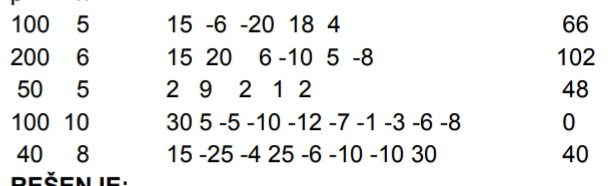# Daily Challenge #72

A restaurant’s got its own garage. An operator works there.
The ramp can be open or closed.
When the ramp is open there can enter 3 cars per minute (if there is space).
When the ramp is closed 2 cars can exit per minute.
Ask for the amount of spaces there are for the cars to park in the garage.
Then, the portion of the cars that entered/left.
The output should be how many cars are in the garage in the end.

Examples: (input1, input2, output)Have fun!

4 Likes

Solution in python:

Summary
``````def negativ(n):
return n<0
[p,n] = map(int, input().split())
minuti = list(map(int, input().split(" ")))
vozila = 0
for elem in minuti:
if negativ(elem):
if negativ(vozila-abs((abs(elem)*-2))):vozila = 0
else:vozila -= abs((abs(elem)*-2))
else:
if vozila+(elem*3) > p:vozila = p
else:vozila+=elem*3
print(vozila)
``````
2 Likes

Hint:

If the car status is negative, that’s how many portions of cars left, and the other way around.

2 Likes

Is that a Math questionjk
btw, it is interesting but I have no computer in my hand.
I can’t do with my phone either

1 Like

My solution in blocks:

The second value in `input1` is entirely redundant, it seems.